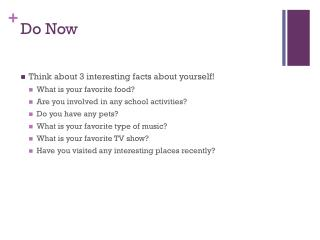DownloadDownload PresentationDo Now

# Do Now

Télécharger la présentation## Do Now

- - - - - - - - - - - - - - - - - - - - - - - - - - - E N D - - - - - - - - - - - - - - - - - - - - - - - - - - -
##### Presentation Transcript

1. Do Now • Think about 3 interesting facts about yourself! • What is your favorite food? • Are you involved in any school activities? • Do you have any pets? • What is your favorite type of music? • What is your favorite TV show? • Have you visited any interesting places recently?

2. Go Over Quizzes

3. 1.7 Completing the Square Objectives: SWBAT solve quadratic equations by completing the square

4. Solve the quadratic equation by finding the square roots x² - 8x + 16 = 25

5. Page 51# 1 and # 2 1. x² + 6x +9 = 36 2. x² - 10x + 25 = 1

6. Completing the square x² + bx

7. Completing the square

8. Completing the square

9. Completing the square

10. Completing the square • How can we right this algebraically?

11. Examples: Make a perfect square trinomial Find the value of c that makes x² + 16x + c a perfect square trinomial. Then write the expression as the square of a binomial.

12. Examples: Make a perfect square trinomial

13. Page 51Find the value of c that makes a perfect square trinomial. Then write the expression as the squareof a binomial.# 4 : #5: x² + 14x + c x² + 22x + c

14. Solving Equations ax² + bx + c = 0 ax² + bx + ___= -c ax² + bx + (b/2) ² = -c+(b/2) ² (ax+b/2) ²= -c+(b/2) ²

15. Example: Solve ax² + bx + c = 0 when a=1 x² - 12x + 4 = 0 x² - 12x + ____ = -4 x² - 12x + (-12/2) ²= -4+(-12/2) ² x² - 12x + 36 = -4+36 (x-6) ² = 32 (x-6) = +-√(32) X=6+-√(32) X=6+4√2 X=6-4√2

16. Solve the equation by completing the squarePage 52 #7) x² +6x + 4 = 0 #8) x² - 10x + 8 =0

17. What about if ax² + bx+ c = 0 and a ≠ 1 2x² + 8x + 14 = 0 x² + 4x + 7 = 0 x² + 4x = -7 x² + 4x + (4/2)²= -7 + (4/2)² x² + 4x + 4= -7+4 (x+2)² = -3 x+2 = √-3 X= -2+-i√3

18. Solve the equation by completing the square page 52 #10. 3x² + 12x - 18=0 #11. 6x(x+8) = 12

19. Home Work • Page 54 • 3-5, 9-11, 14-16, 25-27, 29-31